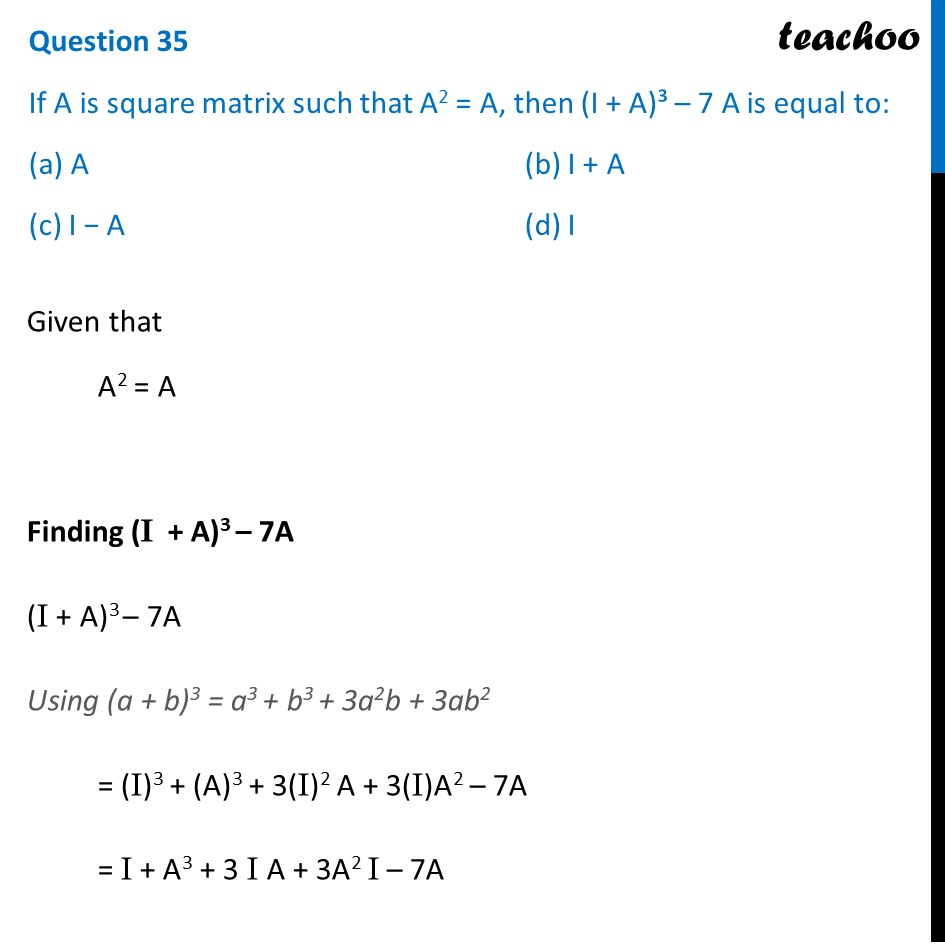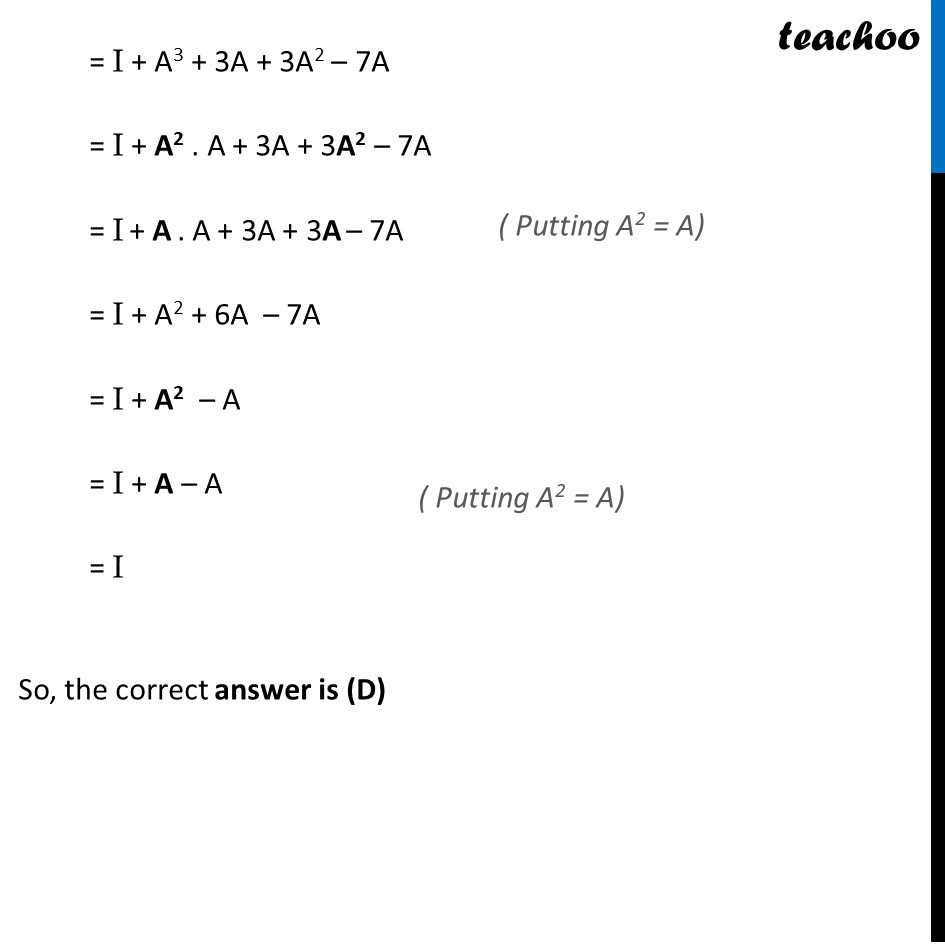CBSE Class 12 Sample Paper for 2022 Boards (MCQ Based - for Term 1)

Class 12
Solutions of Sample Papers and Past Year Papers - for Class 12 Boards

## (c) I − A         (d) I

This question is inspired from Misc. 15 (MCQ) - Chapter 3 Class 12 - MatricesLearn in your speed, with individual attention - Teachoo Maths 1-on-1 Class

### Transcript

Question 35 If A is square matrix such that A2 = A, then (I + A)³ – 7 A is equal to: (a) A (b) I + A (c) I − A (d) I Given that A2 = A Finding (I + A)3 – 7A (I + A)3 – 7A Using (a + b)3 = a3 + b3 + 3a2b + 3ab2 = (I)3 + (A)3 + 3(I)2 A + 3(I)A2 – 7A = I + A3 + 3 I A + 3A2 I – 7A = I + A3 + 3A + 3A2 – 7A = I + A2 . A + 3A + 3A2 – 7A = I + A . A + 3A + 3A – 7A = I + A2 + 6A – 7A = I + A2 – A = I + A – A = I So, the correct answer is (D)